# Lobachevskii space

Jump to: navigation, search

A space whose geometry is defined by the axioms of Lobachevskii geometry. In a wider sense a Lobachevskii space is a non-Euclidean hyperbolic space whose definition is connected with concepts of the geometry of a pseudo-Euclidean space. Let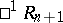be the Lorentz–Minkowskian-space with one time-like direction. A sphere of time-like radius is analogous to a hyperboloid of two sheets. One sheet (say the "future" sheet) is isometric to a Lobachevskii-space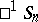. This definition of a Lobachevskii space makes it possible to include this space in the projective classification of non-Euclidean spaces. The spacein the projective spaceis represented by the interior of an oval (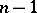)-quadric that is the intersection of an-sphere of time-like radius with the hyperplane at infinity of the spacethat completes this space to the projective space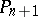. The points of the oval ()-quadric are the points at infinity of, that is, the quadric is the absolute of this space. The outside of this quadric, which completesto the complete space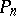, is called the ideal domain of. This interpretation is called the Cayley–Klein projective interpretation. It can also be obtained by projecting an-sphere of time-like radius infrom its centre to a tangent-plane, which is a Euclidean-space; the spaceis represented by the inside of an-ball in this-plane, and the boundary of the-ball is the absolute of(the latter interpretation ofin the Euclidean space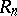is sometimes called the Beltrami–Klein interpretation).

The projective interpretation of Lobachevskii-space makes it possible to verify the axioms of Lobachevskii geometry, to give a representation of all figures of this geometry, and to establish their properties; in particular, in this interpretation it is easy to establish the geometrical properties of the Lobachevskii-plane that follow from the axioms of Lobachevskii geometry.

When the hyperbolic spaceis imbedded in the projective space, an-flat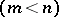is said to be proper if it intersects the absolute in an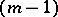-quadric; an-flat that touches the absolute is isotropic; and an-flat that does not intersect the absolute is ideal. The poles of proper hyperplanes are ideal points, and the proper points are the poles of ideal hyperplanes. More generally, the polar (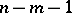)-flats of proper-flats of the Lobachevskii spaceare ideal ()-flats, and the polar ()-flats of ideal-flats are proper ()-flats.

In the space, as coordinates of a pointone takes the components of the corresponding vector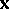of this point in. These Weierstrass coordinates must satisfy the condition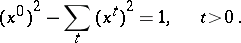Inone may introduce instead coordinates, analogous to spherical polar coordinates, which are connected with the coordinatesby the relations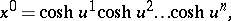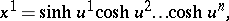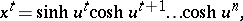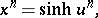The distancebetween two points ofis then defined, in terms of their Weierstrass coordinates, by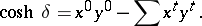The angle(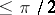) between two intersecting hyperplanesand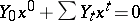(where) can be identified with the space-like distance between their poles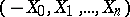and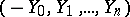, and is thus given by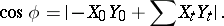Similarly, the distancebetween two ultraparallel hyperplanes is given byThe distance between points and the values of the angles between planes admit expressions in terms of the cross ratios (cf. Cross ratio) of points, using points of the absolute.

In the Lobachevskii spaceone can define spheres (balls), equi-distant surfaces, horospheres (horocycles for, cf. Horocycle),-simplexes, etc.

The classification of motions of the Lobachevskii spaceas collineations that take points of the absolute (oval quadric) into itself reduces to the classification of motions fixing one point of the Lorentz–Minkowskian spacewithout interchanging "future" and "past" . (This is a Lie group.) In order to specify a motion ofit is sufficient to give the images ofpoints that do not lie in one hyperplane.

There are several conformal interpretations of a Lobachevskii space, one of which is the Poincaré model. It is also possible to have a conformal interpretation of the space on one of its hyperplanes. Apart from these there are interpretations in complex spaces. In particular, for the spaceone can construct the Kotel'nikov interpretation of manifolds of lines.

By means of projective interpretations, quadrics in, and particularly in the-plane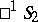, can be classified more completely.

The spaceis a Riemannian-space of constant negative curvature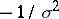, where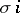is the radius of curvature of the space. The geometry of a Lobachevskii space in sufficiently small neighbourhoods of points is close to the geometry of the Euclidean space of the same dimension.

In the large, the spaceis homeomorphic to the space; it extends indefinitely in all directions. Any-flat of,, is a space. Also, straight lines ofare geodesics, and-flats are totally geodesic-surfaces of this space.

In the projective classification of metrics of non-Euclidean spaces a Lobachevskii space is also classified with respect to the metrics on lines, pencils of planes and-flats. In particular, on a-flat of a Lobachevskii space the projective metric on a line is hyperbolic, and the metric in pencils of lines is elliptic.